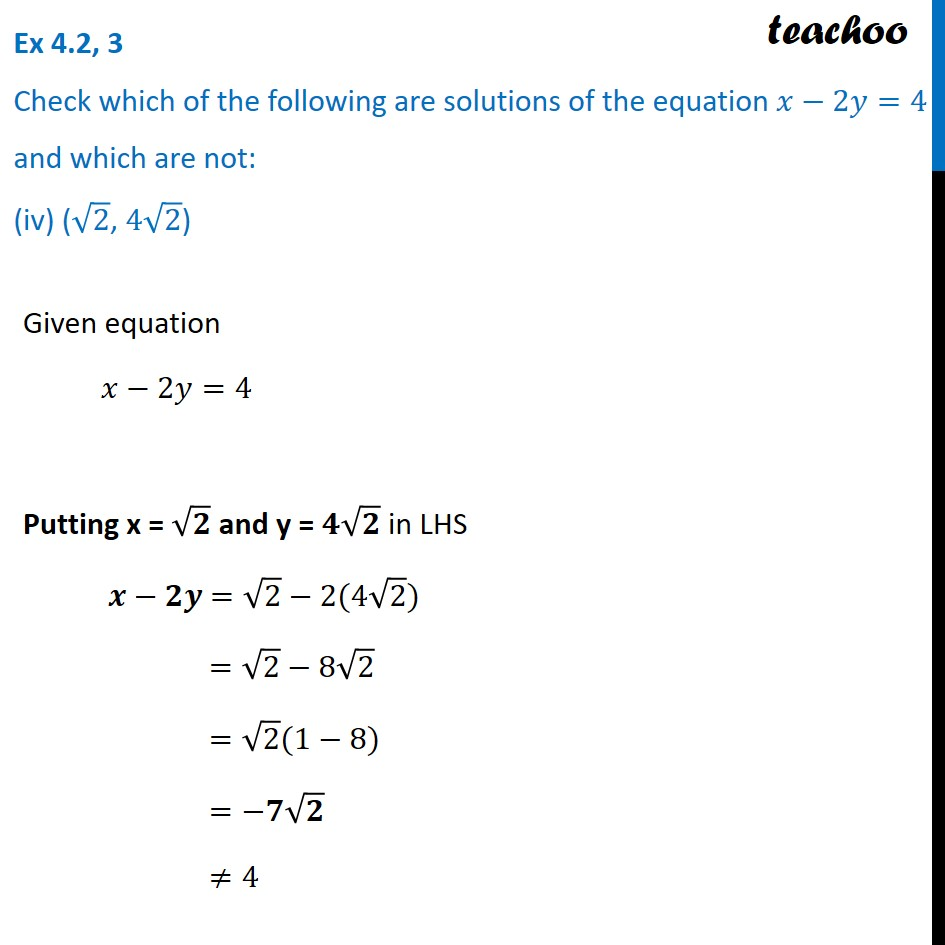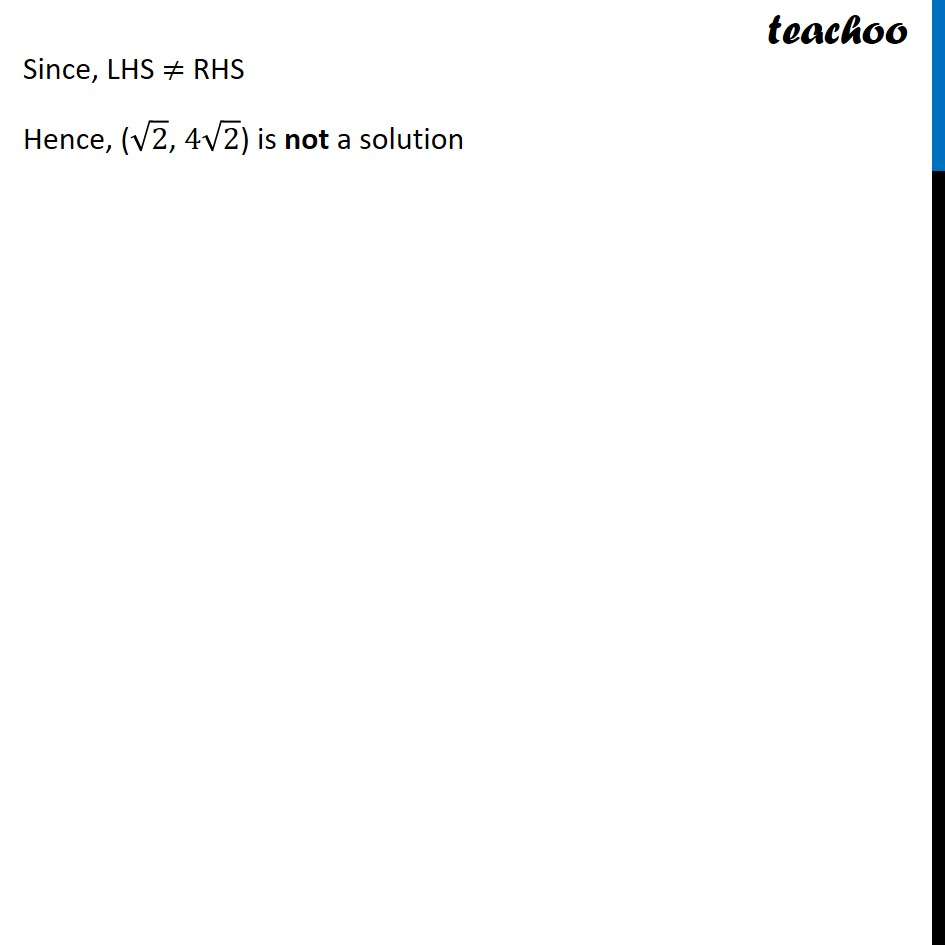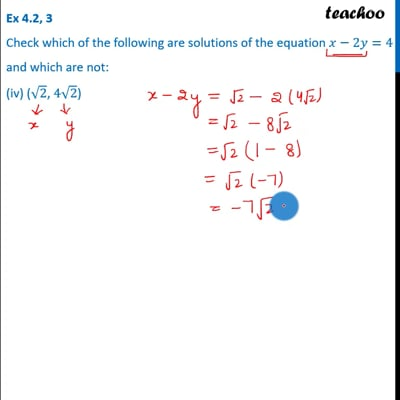Ex 4.2

Chapter 4 Class 9 Linear Equations in Two Variables
Serial order wiseThis video is only available for Teachoo black users

Introducing your new favourite teacher - Teachoo Black, at only ₹83 per month

### Transcript

Ex 4.2, 3 Check which of the following are solutions of the equation 𝑥−2𝑦=4 and which are not: (iv) (√2, 4√2) Given equation 𝑥−2𝑦=4 Putting x = √𝟐 and y = 𝟒√𝟐 in LHS 𝒙−𝟐𝒚 =√2−2(4√2) =√2−8√2 =√2(1−8) =−𝟕√𝟐 ≠4 Since, LHS ≠ RHS Hence, (√2, 4√2) is not a solution